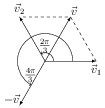# Linear and Angular Simple Harmonic Motion

## Problems from IIT JEE

Problem (IIT JEE 2011): A point mass is subjected to two simultaneous sinusoidal displacements in $x$~direction, $x_{1}(t)=A\sin(\omega t)$ and $x_2(t)=A\sin\left(\omega t+\frac{2\pi}{3}\right)$. Adding a third sinusoidal displacement $x_3(t)=B\sin(\omega t+\phi)$ brings the mass to complete rest. The value of $B$ and $\phi$ are,

1. $\sqrt{2} A$, $3\pi/4$
2. $A$, $4\pi/3$
3. $\sqrt{3} A$, $5\pi/6$
4. $A$, $\pi/3$

Solution: Adding $x_3(t)$ brings the mass to complete rest. Thus, $x_1(t)+x_2(t)+x_3(t)=0$, which gives, \begin{alignat}{2} B\sin(\omega t+\phi)&=-A \left[\sin (\omega t)+\sin \left(\omega t+2\pi/3\right) \right]\nonumber\\ &=-A \left[2\sin\left(\omega t+\pi/3\right) \cos(-\pi/3)\right]\nonumber\\ &=-A\sin\left(\omega t+\pi/3\right)=A\sin\left(\omega t+4\pi/3\right)\nonumber \end{alignat}Aliter: The problem can be solved graphically using vector addition. The displacement $x_1(t)$ can be represented by a vector $\vec{v}_1=A\angle 0$ and displacement $x_2(t)$ by $\vec{v}_2=A\angle (2\pi/3)$ (see figure). The resultant of these two is $\vec{v}=A\angle(\pi/3)$. The resultant of the three is zero if displacement $x_3(t)$ is $-\vec{v}=A\angle(4\pi/3)$.